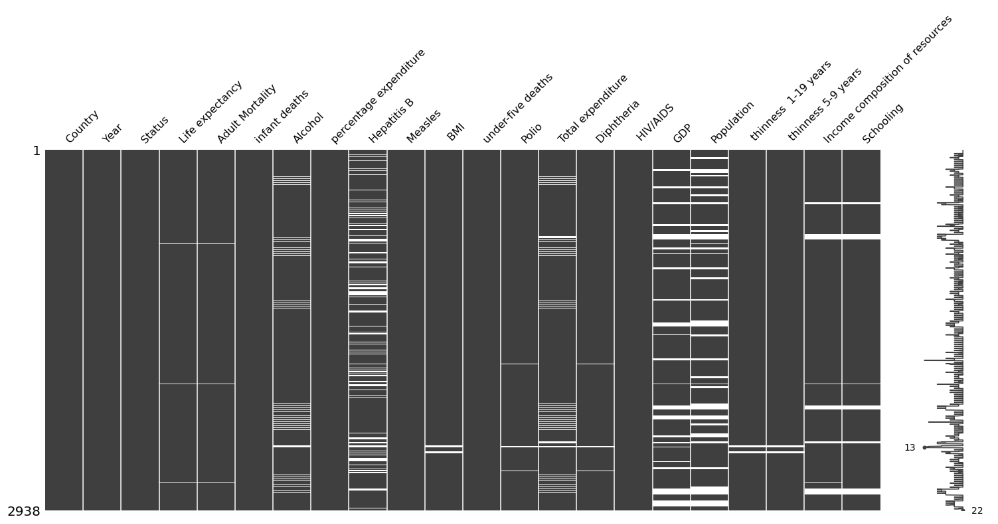Search
•Magdalena Konkiewicz

# Some lesser-known Data Science libraries And how to use them with python code…Image by Gerhard G. from Pixabay

Introduction

Have you been practicing Data Science for a while now? You must know pandas, scikit-learn seaborn, and matplotlib pretty well at this stage.

If you feel like you want to expand your horizons and learn some more obscure libraries but equally useful ones you are in a good place. In this article, I will show you some lesser-known libraries for Data Scientists in python.

Let’s get started.

imbalanced learn

If you have been building some supervised machine learning models in the past you will know that the class imbalance in the target variable can be a big problem. This is caused because by the fact that there are not enough examples in the minority class for the algorithm to learn the pattern.

A solution is to create some synthetic samples that will augment the minority class for learning by using for example SMOTE (Synthetic Minority Over-sampling Technique). Luckily imbalance learn library will help you to implement this technique on any imbalanced data set.

You can install imbalance learn library by running the following command in your terminal.

```
pip install imbalanced-learn

```

In order to demonstrate balancing the data set, we will download a breast cancer data set from the sci-kit learn library.

```
import pandas as pd
df = pd.DataFrame(data.data, columns=[data.feature_names])
df[‘target’] = data[‘target’]

```Now let’s see the distribution of our target variable.

```
df.target.value_counts()

```The data set is definitively note evenly distributed even though it is not terribly imbalanced: we have 357 patients with breast cancer and 212 patients that are healthy. Let’s see if we can make it a bit more balanced. We will oversample the 0 class using SMOTE.

```
from imblearn.over_sampling import SMOTE
oversample = SMOTE()
X_oversample, y_oversample = oversample.fit_resample(data.data, data.target)
pd.Series(y_oversample).value_counts()

```As you can see the data set is perfectly balanced now. We have 357 instances of each class. As a result of our operations, there were 145 artificial instances created.

stats models

This is another great library that has been designed especially building statistical models. I normally use it for fitting linear regression

It is really easy to use and straight away you get a lot of information about the model such as R2 BIC, AIC, coefficients, and their corresponding p-values. This information is more difficult to access when using the linear regression from scikit-learn.

Let’s have a look at how you can fit a linear regression model using this library. Let’s first download a Boston house prices data set.

```
import pandas as pd
df = pd.DataFrame(data.data, columns=[data.feature_names])
df[‘target’] = data[‘target’]

```Above we have the first five rows of our data set. There are thirteen features and we can see that a target variable is a continuous number. This is a perfect data set for regression.

Let’s now install the stats models library using pip

```
pip install statsmodels

```

Now we can try to fit the linear regression model to our data using the following code.

```
import statsmodels.api as sm
model = sm.OLS(df.target, X).fit()
predictions = model.predict(X)
print_model = model.summary()
print(print_model)

```As you can see we have just fitted a linear regression model to this data set and got printed a detailed summary of the model. You can read all the important information really easily, readjust your features if necessary, and rerun the model.

I find it easier to use stats models for regression in comparison to the scikit-learn version due to the fact that all the information I need is presented in this short report.

missingno

Another useful library is missingno. It helps you to visualize the distribution of missing values.

You probably are used to checking for missing values in pandas using isnull() function. This helps you to get the number of missing values for each column but does not help you identify where they are. This is exactly when missingo becomes useful.

You can install the library using the command below:

```
pip install missingno

```

Let’s now demonstrate how you can use missingo to visualize missing data. In order to do it, we will download the life expectancy data set from Kaggle.

You can then load the dataset using read_csv() function and then call matrix() function from missingno library.

```
import pandas as pd
import missingno as msno
msno.matrix(df)

```As a result, you can see where the missing values are located. It is very useful if you suspect that missing values are in some specific location or following some specific pattern.

Summary

In this tutorial, you have learned how to use some lesser-known data science libraries. Specifically, you learned:

• how to balance data set using SMOTE technique and inbalance learn library,

• how to perform linear regression using stats models,

• and how to visualize missing values using missingno.

I hope you can use in practice what you have learned in practice. Happy coding!